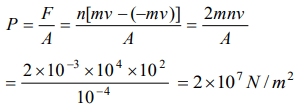## Laws of Motion Questions and Answers Part-12

1. A satellite in force-free space sweeps stationary interplanetary dust at a rate $dM\diagup dt =\alpha v$ where M is the mass, v is the velocity of the satellite and $\alpha$ is a constant. What is the deacceleration of the satellite
a) $-2\alpha v^{2}\diagup M$
b) $-\alpha v^{2}\diagup M$
c) $+\alpha v^{2}\diagup M$
d) $-\alpha v^{2}$

Explanation:2. 10,000 small balls, each weighing 1 gm, strike one square cm of area per second with a velocity 100 m/s in a normal direction and rebound with the same velocity. The value of pressure on the surface will be
a) $2\times 10^{3} N\diagup m^{2}$
b) $2\times 10^{5} N\diagup m^{2}$
c) $10^{7} N\diagup m^{2}$
d) $2\times 10^{7} N\diagup m^{2}$

Explanation:3. Swimming is possible on account of
a) First law of motion
b) Second law of motion
c) Third law of motion
d) Newton's law of gravitation

Explanation: Swimming is a result of pushing water in the opposite direction of the motion.

4. When we jump out of a boat standing in water it moves
a) Forward
b) Backward
c) Sideways
d) None of the above

Explanation: Because for every action there is an equal and opposite reaction takes place.

5. You are on a frictionless horizontal plane. How can you get off if no horizontal force is exerted by pushing against the surface
a) By jumping
b) By spitting or sneezing
c) By rolling your body on the surface
d) By running on the plane

Explanation: By spitting or sneezing

6. On a stationary sail-boat, air is blown at the sails from a fan attached to the boat. The boat will
a) Remain stationary
b) Spin around
c) Move in a direction opposite to that in which air is blown
d) Move in the direction in which the air is blown

Explanation: The force exerted by the air of fan on the boat is internal and for motion external force is required.

7. A man is at rest in the middle of a pond on perfectly smooth ice. He can get himself to the shore by making use of Newton's
a) First law
b) Second law
c) Third law
d) All the laws

Explanation: Third law

8. A cannon after firing recoils due to
a) Conservation of energy
b) Backward thrust of gases produced
c) Newton's third law of motion
d) Newton's first law of motion

Explanation: Newton's third law of motion

9. Newton's third law of motion leads to the law of conservation of
a) Angular momentum
b) Energy
c) Mass
d) Momentum

10. A man is carrying a block of a certain substance (of density $1000 kgm^{-3}$  ) weighing 1 kg in his left hand and a bucket filled with water and weighing 10 kg in his right hand. He drops the block into the bucket. How much load does he carry in his right hand now